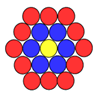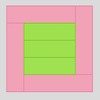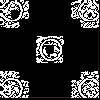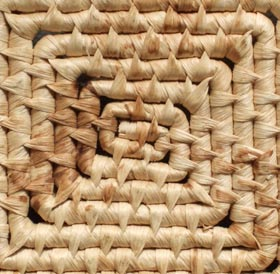#### You may also like### Counting Counters

Take a counter and surround it by a ring of other counters that MUST touch two others. How many are needed?### Cuisenaire Squares

These squares have been made from Cuisenaire rods. Can you describe the pattern? What would the next square look like?### Doplication

We can arrange dots in a similar way to the 5 on a dice and they usually sit quite well into a rectangular shape. How many altogether in this 3 by 5? What happens for other sizes?

# Rope Mat

## Rope Mat

I have a square table-mat made from rope that spirals from the centre. It is rather like this one:The mat is a $14$ centimetre square. The rope is $2$ cm wide.

How many centimetres of rope will I need to make another mat just like it?

How much rope would I need to make a $12$ cm square, or a $16$ cm square?

Is there a quick way to work this out?

### Why do this problem?

This problem requires an understanding of length and confidence to use calculations, whether multiplication, division, addition or subtraction. It is a good context in which to highlight how useful it can be to to draw a diagram. It also provides an opportunity for learners to consider the effectiveness of alternative strategies.

### Possible approach

You could start by showing the group a mat that coils out from the centre, even if it is the more usual circular kind, asking how they think a square mat would be made. You could ask them to imagine a square mat which was quite small, say $6$ cm by $6$ cm, and challenging them to work out how long the rope would need to be. Alternatively/in addition, you could ask what size of mat could be made from a piece of rope which was $8$ cm long.

Having familiarised the class with the context of the problem, you could invite them to work in pairs on the main task so that they are able to talk through their ideas with a partner. Have plenty of squared paper available ($1$ cm squares would be ideal) but resist forcing pairs to use it! They may come up with alternative ways of representing the problem which will be worth sharing in the plenary. Mini-whiteboards and/or plain paper would also be useful.

When the initial part of the problem has been done and possible methods of reaching the answer discussed, it could be the right time to look at the final part of the problem. Can learners come up with a quick way to calculate the length of rope needed for any sized square mat?

### Key questions

Where will the rope coil next?
Can you think of another way of working it out?
Can you see why your calculation works?

### Possible extension

Learners could not only investigate different sizes of square mat, as in the problem, but also different thicknesses of rope.

### Possible support

It might be useful to have the final square, $14$ cm sides, already drawn on squared paper ($1$ or $2$ cm squares would be best) for some children to use. They may need to experiment with finding the centre and then the rope can be drawn coiling round from there.